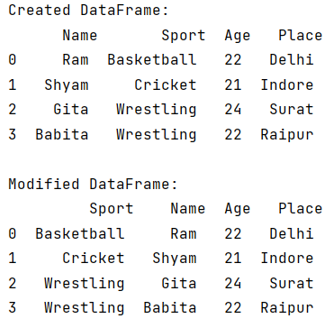# Move column by name to front of table in pandas

Given a Pandas DataFrame, we have to move column by name to front of table.
Submitted by Pranit Sharma, on June 29, 2022

Pandas is a special tool that allows us to perform complex manipulations of data effectively and efficiently. Inside pandas, we mainly deal with a dataset in the form of DataFrame. DataFrames are 2-dimensional data structures in pandas. DataFrames consist of rows, columns, and the data.

Suppose we have a DataFrame having 5 columns named one, two, three, four, and five and you have to shift the column three at the first position, for this purpose, we will first make a list of those values whose columns we want to move, then we will drop that particular column and we will again insert this column at the index 0.

To work with pandas, we need to import pandas package first, below is the syntax:

```import pandas as pd
```

Let us understand with the help of an example,

## Python code to move column by name to front of table in pandas

```# Importing pandas package
import pandas as pd

# Importing numpy package
import numpy as np

# Creating a DataFrame
df = pd.DataFrame({
'Name':['Ram','Shyam','Gita','Babita'],
'Age':[22,21,24,22],
'Place':['Delhi','Indore','Surat','Raipur']
})

# Display DataFrame
print("Created DataFrame:\n",df,"\n")

# Creating a list of reordered column names
values = df['Sport']

# Dropping this column
df = df.drop(labels=['Sport'],axis=1)

# Inserting these values at the starting
df.insert(0, 'Sport', values)

# Display modified DataFrame
print("Modified DataFrame:\n",df)
```

Output:Languages: » C » C++ » C++ STL » Java » Data Structure » C#.Net » Android » Kotlin » SQL
Web Technologies: » PHP » Python » JavaScript » CSS » Ajax » Node.js » Web programming/HTML
Solved programs: » C » C++ » DS » Java » C#
Aptitude que. & ans.: » C » C++ » Java » DBMS
Interview que. & ans.: » C » Embedded C » Java » SEO » HR
CS Subjects: » CS Basics » O.S. » Networks » DBMS » Embedded Systems » Cloud Computing
» Machine learning » CS Organizations » Linux » DOS
More: » Articles » Puzzles » News/Updates